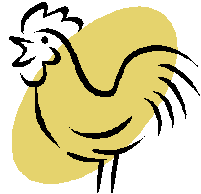# Exam-Style Questions.

## Problems adapted from questions set for previous Mathematics exams.

### 1.

GCSE Higher

Strat drove from Wolverhampton to London via Coventry.

• The distance from Wolverhampton to Coventry is 56 km.
• The distance from Coventry to London is 156 km.
• Strat's average speed from Wolverhampton to Coventry was 63 km/h.
• Strat took 90 minutes to drive from Coventry to London.

(a) Work out Strat's average speed for his total drive from Wolverhampton to London.

Raven drove from Bedrock to Springfield via South Park.

• Raven's average speed from Bedrock to South Park was 70 km/h.
• Her average speed from South Park to Springfield was 50 km/h.

Raven says that the average speed from Bedrock to Springfield can be found by working out the mean of 70 km/h and 50 km/h.

(b) If Raven is correct, what does this tell you about the two parts of Raven's journey?

### 2.

GCSE Higher

Mr Finknottle takes his parents for a drive in his car.

He drives the first ten miles in ten minutes then drives at an average speed of 68 miles per hour for 1 hour 45 minutes.

He finds this information about his car:

Average Speed (s)$$s \le 65 mph$$$$s\gt 65 mph$$
Miles travelled per gallon6050

Use the information to find how many gallons of petrol he uses during the drive.

### 3.

GCSE Higher

The density of water (at 20°C) is 1.09 g/cm3.

The density of blood is 1.60 g/cm3.

Three litres of water are mixed with four litres of blood for a scientific experiment. Work out the density of the mixture giving your answer correct to 2 decimal places.

### 4.

GCSE Higher

The distance from Wolverhampton to Cambridge is 115 miles.

Lynn drives from Wolverhampton to Cambridge leaving Wolverhampton at 10:30am. She assumes that her average speed will be 55 mph.

(a) Use Lynn's assumption about her average speed to work out her arrival time in Cambridge.

(b) Lynn's speed was actually lower than her assumption. How does this affect the arrival time?

### 5.

GCSE Higher

The following kinematics formula can be used to work out the distance travelled (displacement) of an object travelling with constant acceleration.

$$s = ut + \frac12 at^2$$

where:

• $$s$$ is the distance measured in metres;
• $$t$$ is the time measured in seconds;
• $$u$$ is the initial speed;
• $$a$$ is the acceleration;

(a) Rearrange the formula to make $$u$$ the subject.

(b) What units would be associated with speed in this case?

(c) What units would be associated with acceleration?

### 6.

GCSE Higher

Henrietta sells the eggs that the chickens on her farm lay.

She has 220 chickens and each chicken lays 5 eggs each week.

Henrietta gives each chicken 80 grams of chicken feed each day which costs £5.50 for a 20 kg bag.

Work out the cost of the chicken feed for every box of six eggs she sells.If you would like space on the right of the question to write out the solution try this Thinning Feature. It will collapse the text into the left half of your screen but large diagrams will remain unchanged.

The exam-style questions appearing on this site are based on those set in previous examinations (or sample assessment papers for future examinations) by the major examination boards. The wording, diagrams and figures used in these questions have been changed from the originals so that students can have fresh, relevant problem solving practice even if they have previously worked through the related exam paper.

The solutions to the questions on this website are only available to those who have a Transum Subscription.

Exam-Style Questions Main Page

Search for exam-style questions containing a particular word or phrase:

To search the entire Transum website use the search box in the grey area below.